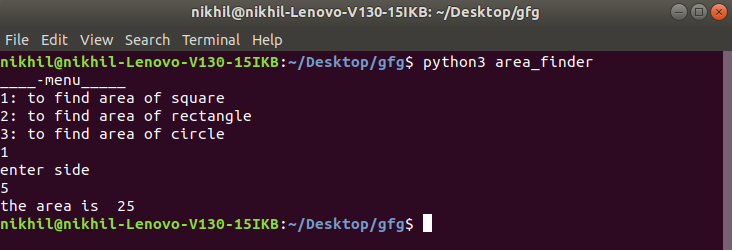Related Articles
Usage of __main__.py in Python
• Last Updated : 10 May, 2020

Many of us have worked with creating their own custom module in Python and is well familiar with the candidate ‘`__init__.py`‘. If you do not know then let’s get a brief and short description of ‘__init__.py’ before diving deep into the concerned topic. Actually, when we try to import a module to Python by default the __init__.py is accessed by Python to look for the necessary imports.

Now there are two scenarios.

• Python files which are well organized to be imported as modules
• Python files (single file structure) which can be run directly by command line

In the first scenario when we try to import it as a module `__init__.py` comes into play. For the second scenario we use some hackish syntax like

 `def` `main ():``    ``pass`` ` `if` `__name__ ``=``=` `'__main__'``:``    ``main()`

This syntax can be related to starting of main in other languages like JAVA and C++. But this syntax will not work ideally with the third scenario. Then what to do if we want to run such files directly from command line ?
This is where __main__.py comes to rescue.

Let’s take an example, we will find the area of a rectangle, square, and circle. For the sake of understanding, let’s divide the three operations under three separate .py files and store it in a module named src.

The file tree will look like this

```+---src
|   |   circle.py
|   |   rectangle.py
|   |   square.py
|   |   __init__.py
|   |
```

Now src can be imported as a module from any other Python program if added to path. But what if we want to run it from command line. For this we store the src folder under a folder named say area_finder and add a file named __main__.py under it . The tree would look like

```area_finder
|   __main__.py
|
+---src
|   |   circle.py
|   |   rectangle.py
|   |   square.py
|   |   __init__.py
|   |
```

content of __main__.py

 `print``(``"____-menu_____"``)``print``("``1``: to find area of square \n\``2``: to find area of rectangle\n\``3``: to find area of circle")`` ` `ch ``=` `int``(``input``())`` ` `if` `ch ``=``=` `1``:``    ``from` `src.square ``import` `square``    ``print``(``"enter side"``)``    ``s ``=` `int``(``input``())``    ``print` `(``"the area is "``, square(s))`` ` `if` `ch ``=``=` `2``:``    ``from` `src.rectangle ``import` `rectangle``    ``print``(``"enter length and breadth"``)``    ``l ``=` `int``(``input``())``    ``b ``=` `int``(``input``())``    ``print``(``"the area is "``, rectangle(l, b))`` ` `if` `ch ``=``=` `3``:``    ``from` `src.circle ``import` `circle ``    ``print``(``"enter radius"``)``    ``r ``=` `int``(``input``())``    ``print``(``"the area is "``, circle(r))`

Now when we run them from terminal/command prompt like this

```python area_finder
```

The output will be –So what happens when we execute the command. Python looks for a file named __main__.py to start its execution automatically. If it doesn’t find it it will throw an error else it will execute main.py and from the code, you can well understand that it will import the modules from src to find the area.

So now we have learned how __main__.py works. Now, let’s take a look at its biggest advantages:

• It removes the ambiguity among end-user about the entry point of the program as Python does it automatically
• It helps in clean execution of the code

Attention geek! Strengthen your foundations with the Python Programming Foundation Course and learn the basics.

To begin with, your interview preparations Enhance your Data Structures concepts with the Python DS Course. And to begin with your Machine Learning Journey, join the Machine Learning – Basic Level Course

My Personal Notes arrow_drop_up